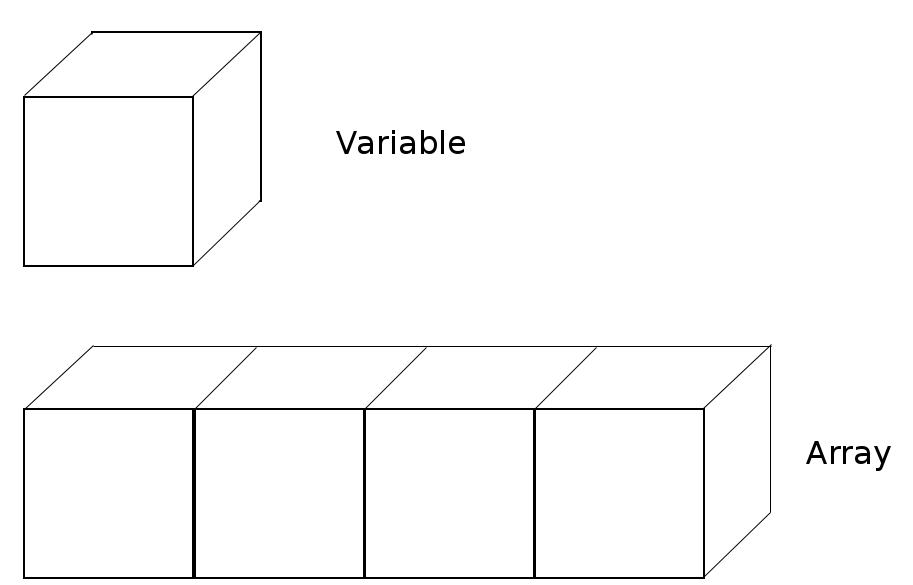# Arrays

An array is a container object that holds values of a same type.Each item in the array is called an element.
Each element has a numeric index, starting with 0.
To access an element, use an index and square brackets, e.g., arr.
Java arrays are also objects.
So, you need to be able to create an array object.

### Examples

```int[] arr = null; //declares an array of integers.

arr = new int;
/*
4 is the size of the array.
It is stored in the variable length of the array and never changes.
JVM initializes all elements of arr to 0.
*/

arr = 1;//assign 1 to 1st element
arr = 2;//assign 2 to 2nd element
arr = 3;//assign 3 to 3rd element
arr = 4;//assign 4 to last element
```

Array literals reduce all the code so far to one line.

```int[] arr2 = {1, 2, 3, 4};
```

Let's create an array that stores references to elements.

```String[] arr3 = null;//declares an array of Strings.
arr3 = new String;//JVM creates the string array and initializes all elements to null.
//You cannot increase or decrease the size of the array.
arr3 = "Java";//1st element is assigned "Java" object’s reference.
arr3 = "School";//2nd element is assigned "School" object’s reference.
```

Array literals allow you to shorten all the code of the above string array to one line.

```String[] arr4 = {"Java", "School"};
```

## How to express strings

To represent strings in code, you need to create a string object from the String class.
If you need the string "Hello", code it like below:

```char[] arrayOfHello = {'H','e','l','l','o'};
String greetings = new String(arrayOfHello);
```

However, most Java programmers use string literals instead of the above code.

```String hello = "Hello";//"Hello" is a String Literal.
```

When JVM calls method main, it creates and passes an array of strings.

```class ArrayTest {
public static void main(String[] args) {
System.out.println(args.length);
}
}
```
C:\ Command Prompt
```C:\javaApp>javac ArrayTest.java

C:\javaApp>java ArrayTest
0
```

Let's make the JVM create an array with size greater than zero.

```class ArrayTest {
public static void main(String[] args) {
System.out.println(args);
System.out.println(args);
System.out.println(args);
}
}
```
C:\ Command Prompt
```C:\javaApp>javac ArrayTest.java

C:\javaApp>java ArrayTest Be The One
Be
The
One
```
```class ArrayTest {
public static void main(String[] args) {
int[] arr = null; //declares an array of integers.
arr = new int;

arr = 1;//assign 1 to 1st element
arr = 2;//assign 2 to 2nd element
arr = 3;//assign 3 to 3rd element
arr = 4;//assign 4 to last element

int[] arr2 = {1, 2, 3, 4};

String[] arr3 = null;//declares an array of Strings.
arr3 = new String;

arr3 = "Java";
arr3 = "School";

String[] arr4 = {"Java", "School"};

char[] arrayOfHello = {'H','e','l','l','o'};
String greetings = new String(arrayOfHello);

String hello = "Hello"; //"Hello" is a String Literal.

System.out.print("arr's length:");
System.out.println(arr.length);

System.out.println("output all elements of arr");
int arrLength = arr.length;
for (int i = 0; i < arrLength; i++) {
System.out.println(arr[i]);
}

System.out.print("arr2's length:");
System.out.println(arr2.length);

System.out.println("output all elements of arr2");
int arr2Length = arr2.length;
for (int i = 0; i < arr2Length; i++) {
System.out.println(arr2[i]);
}

System.out.print("arr3's length:");
System.out.println(arr3.length);
System.out.println("output all elements of arr3");
int arr3Length = arr3.length;
for (int i = 0; i < arr3Length; i++) {
System.out.println(arr3[i]);
}

System.out.print("arr4's length:");
System.out.println(arr3.length);

System.out.println("output all elements of arr4");
int arr4Length = arr4.length;
for (int i = 0; i < arr4Length; i++) {
System.out.println(arr4[i]);
}

System.out.print("output variable greetings:");
System.out.println(greetings);

System.out.print("output variable hello:");
System.out.println(hello);

System.out.print("args's length:");
int argsLength = args.length;
System.out.println(argsLength);

System.out.println("ouput all elements of args");
if (argsLength > 0) {
for (int i = 0; i < argsLength; i++) {
System.out.println(args[i]);
}
}

}

}
```
C:\ Command Prompt
```C:\javaApp>javac ArrayTest.java

C:\javaApp>java ArrayTest Self-pity "D.H. Lawrence"
arr's length:4
output all elements of arr
1
2
3
4
arr2's length:4
output all elements of arr2
1
2
3
4
arr3's length:2
output all elements of arr3
Java
School
arr4's length:2
output all elements of arr4
Java
School
output variable greetings:Hello
output variable hello:Hello
args's length:2
output all elements of args
Self-pity
D.H. Lawrence
```